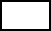Create a new printableMath Worksheets

Sample - Click above to make a new math worksheet (PDF).
 Name _____________________________Date ___________________
Algebra
Simplify.
 1 27 ÷ 9 + 45
 2 90 - 84 ÷ 6
 3 34 ÷ 2 + 40
 4 7 × 2 + 3 - 1
 5 * This is a pre-made sheet.Use the link at the top of the page for a printable page.
 6 54 + 11 - 52 - 1
 7 5 + 37 - 3
 8 61 - 25 + 1 × 31
 9 96 ÷ 3 + 3
 10 90 - 16 ÷ 4
 11 9 × 31 × 20
 12 5 × 35 - 17
 13 79 - 5 + 10 + 2
 14 70 ÷ 7 + 26
 15 85 + 2 - 21

Simplify.
 1 99 × 5 - 13 + 5
 2 40 ÷ 8 + 22 × 1
 3 62 + 45 × 4
 4 7 × 25 - 48
 5 96 ÷ 4 + 3
 6 9 × 24 ÷ 3
 7 64 + 17 × 4
 8 84 ÷ 4 + 25
 9 77 - 4 × 3 - 31
 10 84 + 1 + 26
 11 89 + 3 × 2 - 31
 12 71 - 18 + 1 × 47
 13 7 + 52 × 25 + 2
 14 20 ÷ 4 × 4
 15 56 + 42 ÷ 7

Simplify.
 1 65 - 47 ×  (2 - 45 + 4)
 2 (5 - 1)  +  (40 + 37)
 3 9 +  (1 × 78 ÷ 6)
 4 (80 ÷ 8 × 5)  + 4 × 1
 5 74 - 23 + 27
 6 84 ÷ 7 × 48
 7 (84 × 43)  ×  (4 - 4)
 8 8 + 40 - 1
 9 72 ÷ 8 ×  (28 - 5)
 10 (9 + 35)  + 44 +  (3 + 4)

 Key #2
 aKey #2
Fill in the missing number.
 1- 2 + 54 ÷ 6  =  82
 2×  (20 - 2 - 18)   =  0
 3 96 ÷ 8 -=  9
 4 (6 × 4)  × 23 ×  (- 4)   =  24,288
 5 6 ×  (÷ 3 + 20)   =  276
 6 (8 + 2)  +  (3 ×)   =  13
 7 (54 ÷ 2)  ×  (4 +)  × 21  =  3,969
 8× 45 + 2  =  3,332
 9 (- 90 ÷ 5)   =  68
 10 (9 × 4 + 5 - 2)  ×=  1,560

Complete by evaluating each expression.
1.
 3s - 4

for s = 2
 2 4qfor q = 5
 3 2vfor v = 9
4.
 h9

for h = 63
5.
 k4

for k = 8
6.
 8b - 21

for b = 3

Express each phrase as an algebraic expression.
 1 a number j multiplied by 27
 2 quotient of a number d and 7
 3 sum of 48 and a number u
 4 subtract a number t from 17
 5 a number w increased by 6
 6 40 more than a number g

Solve each equation.
1.
 99  +  x = 130

2.
 68 = p  +  43

3.
 27  +  f = 121

4.
 148 = g  +  82

5.
 b  +  20 = 63

6.
 76  +  w = 91

7.
 181 = v  +  95

8.
 145 = 47  +  t

9.
 147 = 80  +  m

 Key #2
 aKey #2
Solve each equation.
1.
 56 = u  -  26

2.
 28 = 71  -  e

3.
 y  -  48 = 37

4.
 76  -  x = 30

5.
 41 = a  -  33

6.
 88  -  r = 53

7.
 83  -  n = 39

8.
 20 = h  -  64

9.
 21 = d  -  65

Solve each equation.
1.
 12 = 2q

2.
 5 = 25  ÷  c

3.
 6f = 24

4.
 3p = 27

5.
 3 = z  ÷  4

6.
 8k = 16

7.
 56  ÷  b = 8

8.
 7 = f  ÷  9

9.
 x  ÷  4 = 8

Solve each equation.
1.
 p  -  35 = 53

2.
 129 = 85  +  m

3.
 8 = 4d

4.
 3h = 15

5.
 128 = 77  +  t

6.
 4 = 94  -  n

7.
 s  -  15 = 82

8.
 u  +  37 = 46

9.
 y  +  67 = 106

Complete the function table.
1.   Rule: a = e + 26
 Input e 1 2 3 4 5 Output a2.   Rule: c = z + 9
 Input z 6 12 18 24 30 36 42 Output cKey #2
 aKey #2
Complete the function table.
1.   Rule: r = q + 22
 Input q 2 14 18 29 41 54 Output r2.   Rule: v = 6a
 Input a 6 8 10 12 14 16 18 Output vWrite the rule for each function. Write the rule as an equation.
1.
 Rule:Input c 3 6 9 12 15 18 21 Output p 21 42 63 84 105 126 147

Complete the function table and write the rule for each function.
1.
 Rule:Input h 6 16 17 29 34 42Output k 2839 51 56 64 73

Write an equation for each problem. Then solve the equation.
 1 A number divided by 6 is 8.
 2 12 plus a number is 23.
 3 29 minus a number is 13.
 4 The difference between a number and 14 is 64.
 5 24 divided by a number is 12.
 6 A number multiplied by 8 is 80.